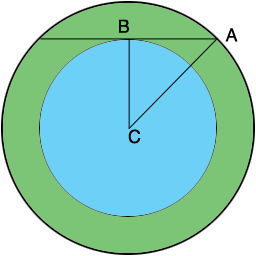SEARCH HOMEMath Central Quandaries & QueriesQuestion from Shannan, a parent: Two concentric circles have radii of 24cm and 26cm. What is the length of the chord that is tangent to the inner circle? Include a sketchHi Shannan,

Did you draw a sketch? In my sketch $C$ is the center of the circle.The important fact here is that since the chord is tangent to the inner circle, angle $CAB$ is a right angle. Thus triangle $ABC$ s a right triangle. You know the lengths of two sides of the right triangle $ABC$ so Pythagoras can tell you the length of the third side.

PennyMath Central is supported by the University of Regina and the Imperial Oil Foundation.